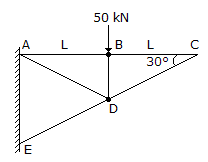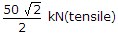# Civil Engineering - UPSC Civil Service Exam Questions

31.

Which one of the following systems of pre-stressing is suitable for pre-tensioned members ?

 A. Freyssinet system B. Magnel-Blaton system C. Hoyer system D. Gifford-Udall system

Explanation:

No answer description available for this question. Let us discuss.

32.

Consider the following statements :
Liquefaction is a phenomenon
1. observed in fine sands
2. associated with development of positive pore pressure.
Which of the statements given above is/are correct ?

 A. 1 only B. 2 only C. Both 1 and 2 D. Neither 1 nor 2

Explanation:

No answer description available for this question. Let us discuss.

33.

Which of the following pairs is/are correctly matched ?
1. Eutrophication.... Nutrient accumulation leading to ecosystem change occurring in impounded water.
2. Autotrophism.... Utilization, rearrangement and decomposition of complex materials predominate.
3. Heterotrophism....Predominance of fixation of light energy, use of simple inorganic substances and built-up complex substances.
Select the correct answer from the codes given below :

 A. 1, 2 and 3 B. 1 alone C. 2 and 3 D. 1 and 3

Explanation:

No answer description available for this question. Let us discuss.

34.

Consider the following statements :
A grillage base is checked for
1. bending
2. shear
3. compression
4. web crippling
Which of these statements are correct ?

 A. 1 and 4 B. 1 and 3 C. 2, 3 and 4 D. 1, 2 and 4

Explanation:

No answer description available for this question. Let us discuss.

35.

In the pin-jointed plane truss shown above, what is the magnitude and nature of force in member BC?A. Zero B. 50 kN (tensile) C. 50 kN (compressive) D.